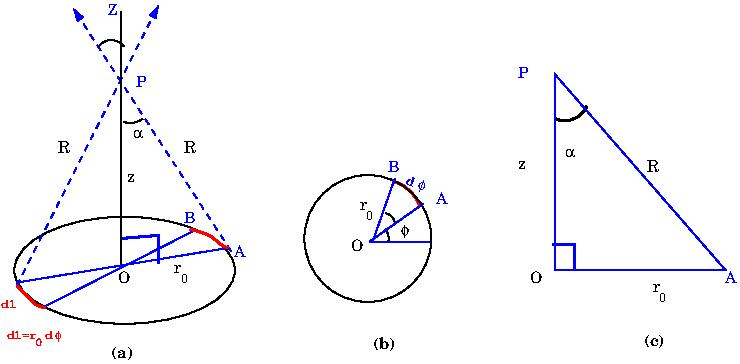# [YMP/EM-02005] Field due to a Uniformly Charged Ring

For page specific messages
For page author info

Category:

The electric field due to a charged ring, at a point on its axis,  is computed using Coulomb's law.

We choose the origin of the coordinate system at the centre of the ring and work with plane polar coordinates $$r,\phi$$. Divide the ring into small elements. One such element, lying in range $$\phi$$ and $$\phi+d\phi$$ is shown in \Figref{Field-Ring}(a) and (b). The radius of the loop is $r_0$. The electric field is to be calculated at a point $$P$$ on the $$Z$$- axis which is taken to coincide with the axis of the loop.

The charge on the line element is $$AB$$ is $$dq=\lambda r_0 d\phi$$. and the electric field  points along $$AP$$ and has magnitude $$dE$$, where \begin{equation} dE = \frac{dq}{4\pi\epsilon_0 R^2} \end{equation} The horizontal component of the field, at the point $$P$$,  due to the ring vanishes. This can be seen by considering contributions from two diametrically opposite line elements as indicated inWe, therefore, need to compute only the $$z$$-component of the electric field.  The $$z$$- component of the field due to the line element $$AB$$ is seen to be \begin{equation} dE_z = dE \cos \alpha =\frac{\lambda dl}{4\pi\epsilon_0 R^2}(\cos\alpha) , \end{equation} and the total field can be written as \begin{eqnarray} {E}_z&=&\int\frac{\lambda dl}{4\pi\epsilon_0 R^2}(\cos\alpha) \label{eq7}, \end{eqnarray}  From the figure we have  $dl=r_0d\phi$,  $\cos\alpha=\frac{z}{R}$ and $R=\sqrt{z^2+r_0^2}$. Therefore

\begin{eqnarray} \nonumber{E}_z&=&\int\frac{\lambda r_0 d\phi}{4\pi\epsilon_0 (z^2+r_0^2)}(cos\alpha)=\frac{\lambda z r_0}{4\pi\epsilon_0 (z^2+r_0^2)^{3/2}}\int d\phi\\ \nonumber&=&\frac{\lambda z r_0}{4\pi\epsilon_0 (z^2+r_0^2)^{3/2}}(2\pi) \\ E_z&=&\frac{qz}{4\pi\epsilon_0(z^2+r_0^2)^{3/2}} \label{eq8} \end{eqnarray} where $q=2\pi r_0\lambda$ is the total charge on the ring. Thus the final answer for the electric field is given by \begin{equation} \vec E = \frac{qz}{4\pi\epsilon_0(z^2+r_0^2)^{3/2}} \, \hat k. \end{equation} For large $$z$$, the field becomes \begin{eqnarray} {E}_z\approx \frac{q}{4\pi\epsilon_0z^2} \label{eq9}. \end{eqnarray}

This is understandable because the ring would appear to be point like and  the entire  charge  $$q$$ on the ring appears to  concentrated at a single point.

One important point, not to be missed, is that one must remember that 'large', or 'small',  always means compared to some quantity. In the present case large $$z$$  means \eqref{eq9} holds for $$z>>r_0$$.

n
0

X